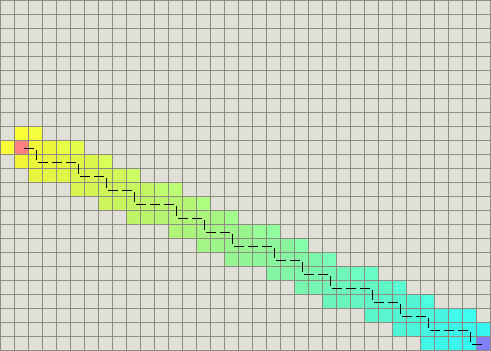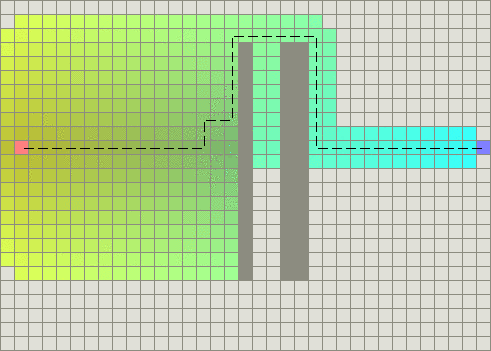#### 栅格地图的启发函数

##### 曼哈顿距离

h(n) = D * (abs(n.x-goal.x) + abs(n.y-goal.y))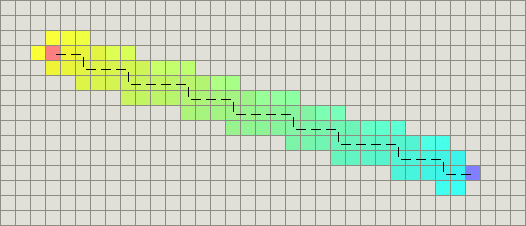(注:上面的图像中,启发函数可以找到好几条最优路径(下文称之为平局).)

##### 对角线距离

h(n) = D * max(abs(n.x-goal.x), abs(n.y-goal.y))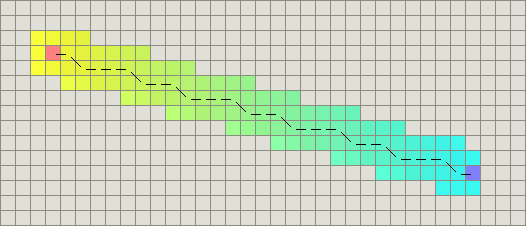h_diagonal(n) = min(abs(n.x-goal.x), abs(n.y-goal.y))
h_straight(n) = (abs(n.x-goal.x) + abs(n.y-goal.y))
h(n) = D2 * h_diagonal(n) + D * (h_straight(n) - 2*h_diagonal(n)))

##### 欧几里得距离

h(n) = D * sqrt((n.x-goal.x)^2 + (n.y-goal.y)^2)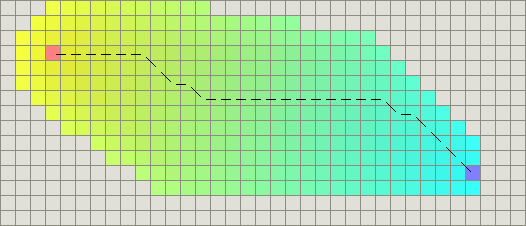##### 平方的欧几里得距离

h(n) = D * ((n.x-goal.x)^2 + (n.y-goal.y)^2)

Do not do this!这绝对会导致g和h的比例的问题.当A*算法计算f(n)=g(n)+h(n)的时候,h的平方会导致它远大于g,这意味着你得到的会是过高估计的启发函数.对于更大的h,这会使得g(n)变得无足轻重了,此时A*算法退化成宽度优先搜索.##### 打破平局f值相同导致的平局

heuristic *= (1.0 + p)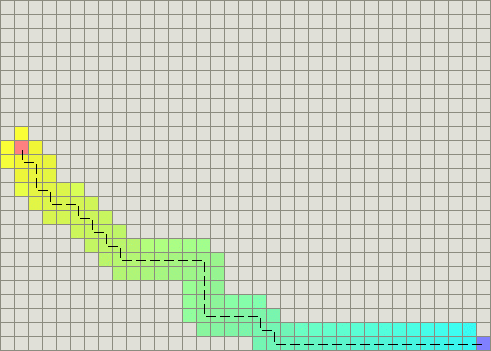Steven van Dijk 提议了一种更为直接的方法,即把h传递给比较函数.当结点的f值是相同的时候,比较函数可以通过h值判定结点优先级来打破平局.

dx1 = current.x - goal.x
dy1 = current.y - goal.y
dx2 = start.x - goal.x
dy2 = start.y - goal.y
cross = abs(dx1*dy2 - dx2*dy1)
heuristic += cross*0.001